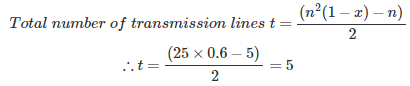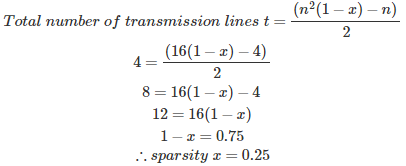# MCQs on Power Systems

##### Page 5 of 67. Go to page 1 2 3 4 5 6 7 8 9 10 11 12 13 14 15 16 17 18 19 20 21 22 23 24 25 26 27 28 29 30 31 32 33 34 35 36 37 38 39 40 41 42 43 44 45 46 47 48 49 50 51 52 53 54 55 56 57 58 59 60 61 62 63 64 65 66 67
01․ Advantages of gauss siedel method is/are
calculation time for each iteration is less
number of iterations are less
applicable for large power system network
all of the above

Advantages of gauss siedel method: 1. It is a simple algebraical equation, so that calculation time for each iteration is less. 2. More suitable for small size networks. Disadvantages of gauss siedel method: 1. More number of iterations are required, so that it has slow convergence. 2. Initial approximate guessing value is required for convergence. 3. It required accelerating factor for convergence. 4. The choice of slack bus affects the convergence. 5. It is not applicable for the large power system networks.

02․ For load flow studies, What are the quantities specified at load bus are
P and V
P and Q
V and δ
δ and Q

Load bus: Known quantities - P and Q Unknown quantities - V and δ Generator bus: Known quantities - P and V Unknown quantities - Q and δ Slack or reference bus: Known quantities - V and δ Unknown quantities - P and Q

03․ Normally Z bus matrix is a
null matrix
sparse matrix
full matrix
unit matrix

Y bus matrix is a sparse matrix, containing more number of zero elements. So that faster calculation is possible. The Y bus matrix is used for the load flow studies. Z bus algorithm or matrix is used for the fault analysis. Inverse of Y bus matrix gives the Z bus matrix, But Z matrix is a full matrix even though Y bus is a sparse matrix. More time is required for inverse of Z bus matrix if the size of the matrix is more than three. But a practical system network has large number of buses and hence Z bus matrix is not uses for the load flow analysis, Y bus is preferred.

04․ For n bus power system size of Y bus matrix is
(n-1)×(n-1)
(n-2)×(n-2)
n×n
(n-1)×(n-2)

Properties of Y bus matrix: 1. Y bus is a square matrix. 2. For n bus power system size of Y bus matrix is n×n 3. Value of diagonal element corresponding to bus i, then Yii = Sum of the admittances connected to bus i. 4. The value of off diagonal elements Yij = Yji , which is connected between bus i and bus j and which is represented with negative sign. 5. Sum of the elements of row i equal to shunt admittances connected to bus i. If this summation is zero, indicates there is no shunt admittance and mutual coupling between the transmission lines.

05․ Which of the following matrix is used for load flow studies?
Y bus matrix
Z bus matrix
Unit matrix
null matrix

Y bus matrix is a sparse matrix, containing more number of zero elements. So that faster calculation is possible. The Y bus matrix is used for the load flow studies. Z bus algorithm or matrix is used for the fault analysis. Inverse of Y bus matrix gives the Z bus matrix, But Z matrix is a full matrix even though Y bus is a sparse matrix. More time is required for inverse of Z bus matrix if the size of the matrix is more than three. But a practical system network has large number of buses and hence Z bus matrix is not uses for the load flow analysis, Y bus is preferred.

06․ Sum of the elements of row i equal to shunt admittances connected to bus i. If this summation is zero, indicates there is no
mutual coupling
both 1 and 2
none of the above

Properties of Y bus matrix: 1. Y bus is a square matrix. 2. For n bus power system size of Y bus matrix is n×n 3. Value of diagonal element corresponding to bus i, then Yii = Sum of the admittances connected to bus i. 4. The value of off diagonal elements Yij = Yji , which is connected between bus i and bus j and which is represented with negative sign. 5. Sum of the elements of row i equal to shunt admittances connected to bus i. If this summation is zero, indicates there is no shunt admittance and mutual coupling between the transmission lines.

07․ A network containing 50 buses in which 10 are the voltage control buses, 6 are the generator buses. Find the size of the Jacobian matrix?
84*84
83*83
34*34
33*33

Size of the Jacobian matrix = (2n-m-2)*(2n-m-2) Where, n = Total number of buses m = Total number of PV buses m = Voltage control buses + Reactive power support buses + generator buses except slack bus Size of the Jacobian matrix = (2*50 -15 - 2)*(2*50 - 15 -) =83*83

08․ If sparsity of a 5 bus transmission line is 0.4. Find the number of transmission lines?
6
5
4
3

n = number of buses x = sparsity09․ A 4 bus power system consists of 4 transmission lines, then find the sparsity of Y bus matrix?
0.25
0.5
0.75
0.4Where, n = number of buses x = sparsity

10․ The value of off diagonal elements is
which is connected between bus i and bus j with negative sign
which is connected between bus i and bus j with positive sign
sum of admittances connected at bus i
sum of admittances connected at bus j

Properties of Y bus matrix: 1. Y bus is a square matrix. 2. For n bus power system size of Y bus matrix is n×n 3. Value of diagonal element corresponding to bus i, then Yii = Sum of the admittances connected to bus i. 4. The value of off diagonal elements Yij = Yji , which is connected between bus i and bus j and which is represented with negative sign. 5. Sum of the elements of row i equal to shunt admittances connected to bus i. If this summation is zero, indicates there is no shunt admittance and mutual coupling between the transmission lines.

<<<34567>>>